## Remove Apostrophe or Text Indicator

This post will guide you how to remove the apostrophe (‘) from the text values in your worksheet in excel. How do I remove text indicator from a text string in a cell in excel. How to use an excel formula to remove text indicator quickly. How to remove leading Apostrophe from a text value in a cell.

## 1. Remove Apostrophe with Excel Formula

If you want to remove the text indicator (‘) or the leading Apostrophe from a text string in one cell, you can create a formula based on the VALUE function.Just like this:

``=VALUE(B1)``

You just need to type this formula into the formula box of the cell C1, then press Enter key in your keyboard. And drag the AutoFill Handler over other cells to apply this formula.

Let’s see the result:

## 2. Remove Apostrophe with Text to Columns Function

You can also use the Text to Columns function to remove the text indicator from text values in one cell, just do the following steps:

Step1: select the cells that you want to remove the Apostrophe or text indicator, such as: B1:B3

Step2: go to DATA tab, click Text to Columns command under Data Tools group. And the Convert Text to Columns Wizard dialog will appear.

Step3: select the Delimited radio button, and then click Finish button.

Step4: you will see the text indicators have been removed.

## 3. Video: Remove Apostrophe or Text Indicator in excel

This video will demonstrate how to remove apostrophes or text indicators in Excel using either a formula or the text-to-columns feature.

## 4. Related Functions

• Excel VALUE function
The Excel VALUE function converts a text value that represents a number to a numeric value.The syntax of the VALUE function is as below:= VALUE  (text)…

## Assigning Points based on Late Time

It is shown in this lesson how to utilize the IF function in Excel to allocate points based on the amount of time that has passed.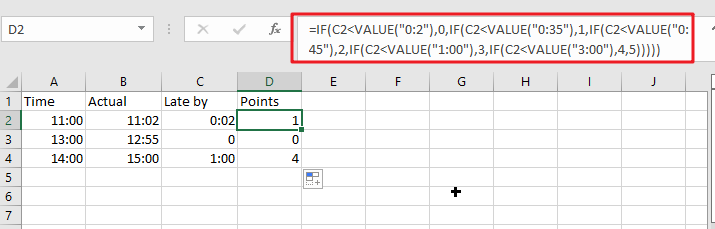## Generic formula:

`=IF(C2<VALUE("0:2"),0,IF(C2<VALUE("0:35"),1,IF(C2<VALUE("0:45"),2,IF(C2<VALUE("1:00"),3,IF(C2<VALUE("3:00"),4,5)))))`

## Return value

This formula provides the number of points that have been assigned depending on the late time.

## How this formula work

For example, below is a list of point regulations and time records; kindly use the formula below to find the appropriate rule or record.

`=IF(C2<VALUE("0:2"),0,IF(C2<VALUE("0:35"),1,IF(C2<VALUE("0:45"),2,IF(C2<VALUE("1:00"),3,IF(C2<VALUE("3:00"),4,5)))))`

A pop-up with further information will appear if you press the Enter key.

To use this formula, just drag the fill handles over the cells.

##IF function is being used to evaluate for defined criteria and then returns the results that match those circumstances. Explain the formula in five parts, as follows:

`IF (late_by_time<VALUE ("0:2"),0,` It returns 0 if the late time is much less than 2 minutes.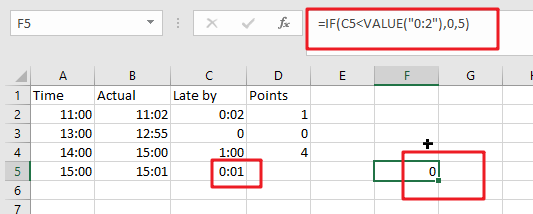`IF (late_by_time <VALUE ("0:35"),1,` The function returns 1 if the late time is much less than 35 minutes but higher than or equivalent to 2 minutes.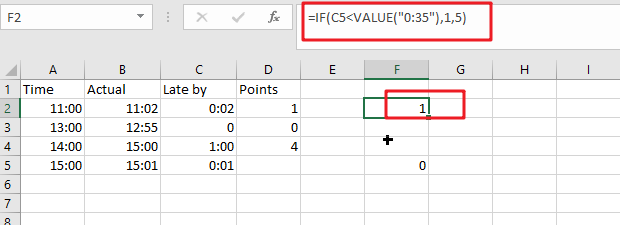`IF (late_by_time <VALUE ("0:45"),2`, If the late time is even less than 45 minutes but higher than or equivalent to 35 mins, it returns 2.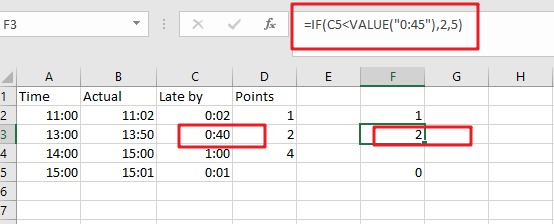`IF (late_by_time <VALUE ("1:00"),3`, If the late time is even less than 60 minutes but higher than or equivalent to 45 mins, it returns 3.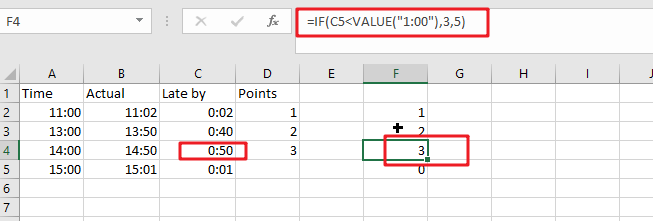`IF (late_by_time <VALUE ("3:00"),4`, The result is 4 if the late time is less than 3 hours but larger than or equivalent to 60 minutes.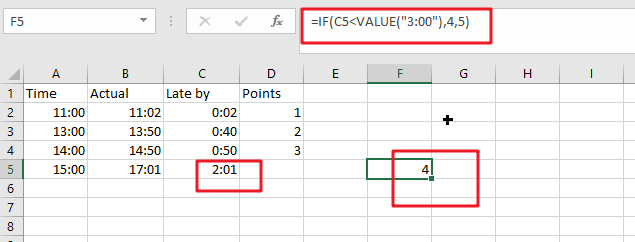5))))) This function returns five if the late time is more than or equal to three hours.

When converting text to numbers, the value function is employed. It does this by converting the text time to a number.

### Related Functions

• Excel IF function
The Excel IF function perform a logical test to return one value if the condition is TRUE and return another value if the condition is FALSE. The IF function is a build-in function in Microsoft Excel and it is categorized as a Logical Function.The syntax of the IF function is as below:= IF (condition, [true_value], [false_value])….
• Excel VALUE function
The Excel VALUE function converts a text value that represents a number to a numeric value.The syntax of the VALUE function is as below:= VALUE  (text)…

## How to Convert Text to Number in Excel

This post will guide you how to convert text or letters to number in Excel. How do I convert text to number with a formula in Excel. How to convert text-based numbers to numeric value in Excel.

Assuming that you have a list of data in range B1:B4, which contain some numbers stored as text in your worksheet. And you need to convert those text-based numbers to numeric value for calculation when using SUM or other Functions. Here are the methods that you can use to convert text into a number.

## Convert Text to Number with a Formula

If you want to convert text values to numbers, you can use a formula based on the VALUE function. Or you can also add zero for text values. The formula is as below:

=VALUE(B1)

Or

=B1 +0

Type this formula into a blank cell and press Enter key on your keyboard. And then drag the AutoFill handle over to other cells to apply this formula.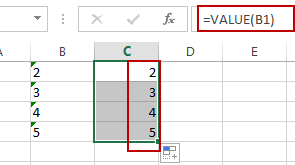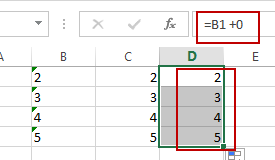Note: The VALUE function will try to convert a number stored as text to a true number. So this formula will return a numeric value.

## Convert Text to Number with Convert to Number Option

When you add one apostrophe into a number, and it will convert the number formula as text format. And you should notice that there is a green triangle at the top left part of the cell.

If you want to convert text to number again, just do the following steps:

#1 select the range of cells that you want to convert.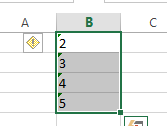#2 click on the yellow diamond shape icon, and select Convert to Number from the drop down menu list.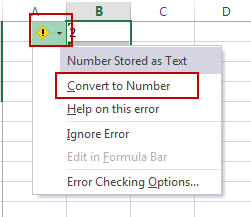You should see that all text values in the selected range have been converted to numbers back.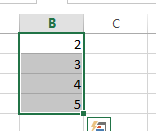## Convert Text to Number with Format Cell Function

If the numbers are formatted as text format, and you can also use the Format Cell function to convert test format to number format easily. Just do the following steps:

#1 select the range of cells that you want to convert.#2 right click on it, and select Format Cell from the drop down menu list. And the Format Cells dialog will open.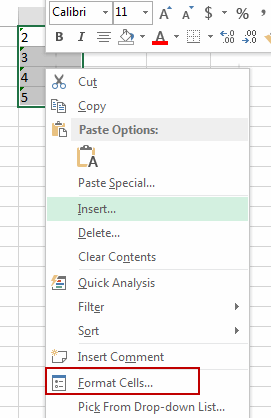#3 Select “Number” tab, then you can select Number category in the category ListBox. The formatting result will be appear on the “Sample” section on the “Format Cells” window. Click Ok button.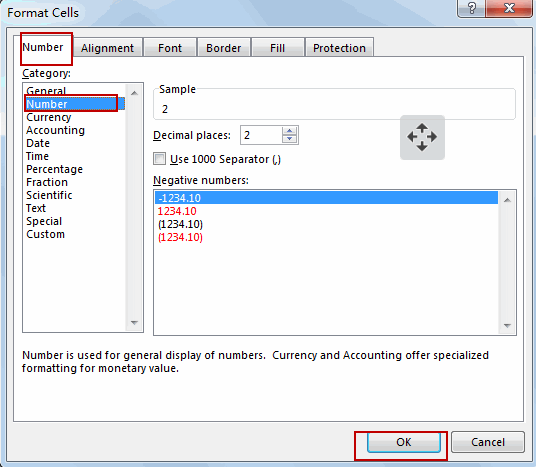Or you can go to HOME tab, click Number command, and select General from the Number Format drop down list.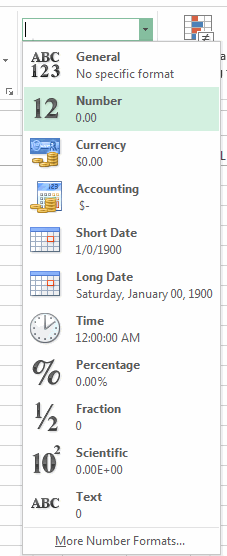This would change the format of the selected cells to general numbers.  And you can also change the number category to other formats, such as: Currency, Accounting, etc.

## Convert Text to Number with Paste Special

You can also use Paste Special Option to achieve the same result of converting text to numbers in Excel. Here are the steps:

#1 Enter number 1 in an empty cell in your worksheet, and you should make sure that it is formatted as number. And press Ctrl + C to copy this cell that contains 1.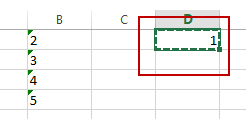#2 select the range of cells that you want to convert its to numbers.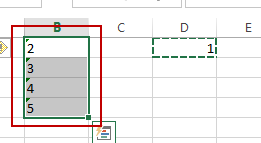#3 right click on it, and select Paste Special from the popup menu list.  And the Paste Special dialog will appear.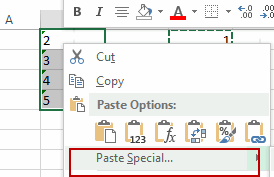#4 select Multiply radio button under Operation section. And click Ok button.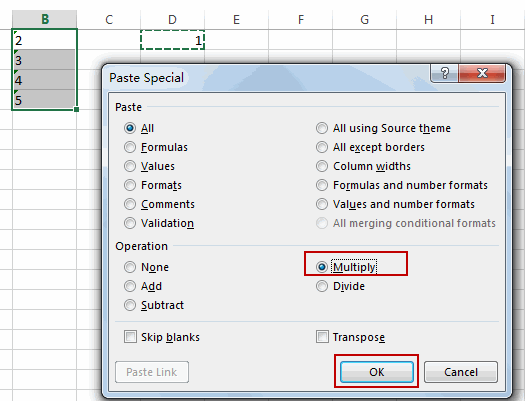#5  you would notice that the text values in the selected range have been converted to numbers.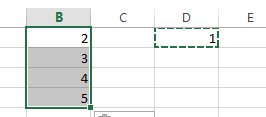## How to keep or remove leading zeros

In the previous post, we talked that how to add leading zeros in the front of a number of text string. And this post explains that how to keep leading zeros as you type in excel. How to prevent automatic truncation of leading zeros in excel. How to remove or delete the leading zeros.

Assuming that you have a list of zip codes in your worksheet, and when you type the zip codes that contains leading zeros, the excel will truncate the leading zeros automatically by default. Such as, you try to type a zip code like “00010086” in a cell, and it will change to “10086”.

If you want to keep the leading zeros as you type in a cell, you need to change the cell format to Text before you type the zip code number. Let’s refer to the following steps:

1# select the range of cells that you want to type the zip codes that contain leading zeros.

2# right click on the selected range, select “Format Cells” from the pop-up menu. The Format Cells window will appear.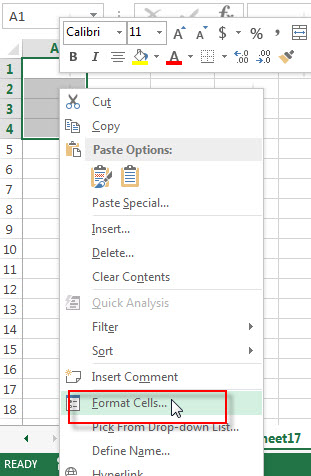3# switch to the Number tab, then choose Text from the Category list.  Click OK button.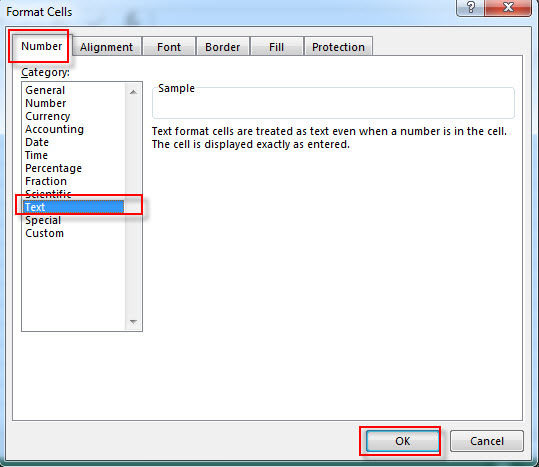4# let’s see the result.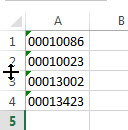Note: you will see a small green triangle in the top-left corner of the cells with leading zeros, it indicates there are some errors with the cell contents. If you want to remove it, you need to select the cells, then click the warning sign, next to click “Ignore Error” from the pop-up menu.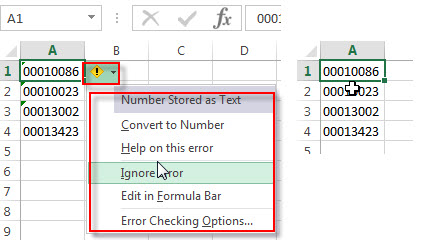There is another way to keep leading zeros as you type, you just need to insert an apostrophe in front of the number you type.  And the apostrophe character will not be displayed in the cell, but excel will treat the contents of the cell as text format.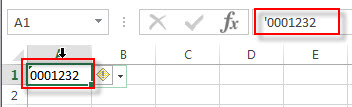If you have a list of phone number with leading zeros, and all numbers are treated as Text format by Excel. if you want to remove all leading zeros in the front of the phone number in a cell, you just need to select the cell,  click exclamation point, then select “Convert to Number” from the pop-up menu.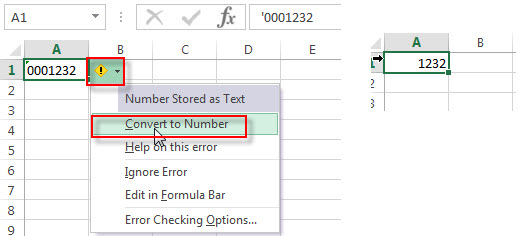Or you can use the VALUE function to convert the text to number.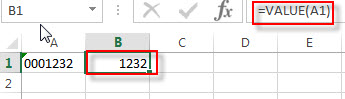## Remove the Leading zeros using VBA macro

You can remove or delete leading zeros in front of the cells with excel VBA macro, just refer to the below steps:

1# click on “Visual Basic” command under DEVELOPER Tab.2# then the “Visual Basic Editor” window will appear.

3# click “Insert” ->”Module” to create a new module4# paste the below VBA code into the code window. Then clicking “Save” button.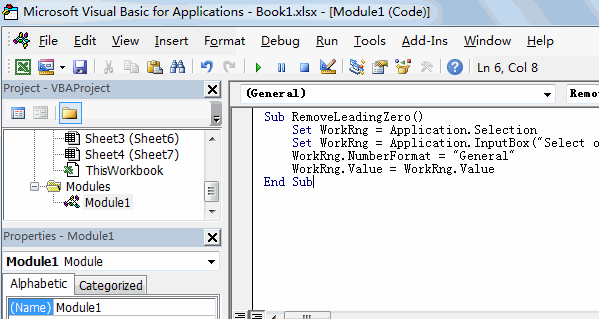```Sub RemoveLeadingZero()
Set WorkRng = Application.Selection
WorkRng.NumberFormat = "General"
WorkRng.Value = WorkRng.Value
End Sub```

5# back to the current worksheet, then run the above excel macro, select the range that you want to delete leading zero.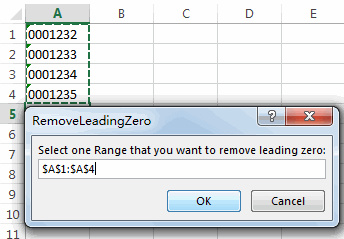6# click “OK” button, you will see that all leading zeros have been removed in the selected range.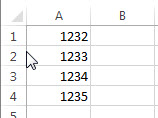### Related Functions

• Excel VALUE function
The Excel VALUE function converts a text value that represents a number to a numeric value.The syntax of the VALUE function is as below:= VALUE  (text)…
• Excel Text function
The Excel TEXT function converts a numeric value into text string with a specified format. The TEXT function is a build-in function in Microsoft Excel and it is categorized as a Text Function. The syntax of the TEXT function is as below: = TEXT (value, Format code)…

### Related Posts

• How to insert leading zeros to number or text
Assuming that you want to insert leading zeros  to number in Cell A2, then you can try to use the TEXT function to create an excel formula. You can also use the CONCATENATE function to add the specific digit of leading zeros into each number…
• Count Numbers with Leading Zeros
Suppost you have a list of product ID in your worksheet, and the product ID contains the different number of leading zeros in the front of string. how to preserve the leading zeros while counting numbers. And you can use another Excel function named as SUMPRODUCT.…

## How to Convet Text Date dd/mm/yy to mm/dd/yyyy as Date Format

This post explains that how to convert text date in the format dd/mm/yy to the standard date format mm/dd/yyyy in excel. How to change dates in text format dd/mm/yy to mm/dd/yyyy as date format in excel 2013.

## Convet Text dd/mm/yy to mm/dd/yyyy as Date Format

If you want to convert dates in the text format to the date format as mm/dd/yyyy, you can use a combination of the DATE function, the VALUE function, the RIGHT function, the MID function, and the LEFT function to create a new excel formula.

For example, to change dates in text format in Cell B1 to a true date format as mm/dd/yyyy (it is a standard date format in excel), you can try to run the following formula in Cell C1:

`=DATE(VALUE(RIGHT(B1,2))+2000), VALUE(MID(B1,4,2)), VALUE(LEFT(B1,2)))`

Let’s see how this formula works:

=VALUE()

The VALUE function converts a text string that represents a number to a numeric value. So this formula will convert text string returned by RIGHT, MID and LEFT functions to a numeric value.

=VALUE(RIGHT(B1,2))+2000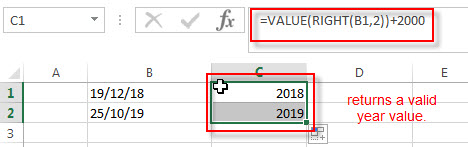The RIGHT function will extract the rightmost 2 characters from text string in Cell B1, then goes into the VALUE function to convert it as a numeric value, then add 2000 to get a valid year value. This year value will go into the DATE function as its year argument.

=VALUE(MID(B1,4,2))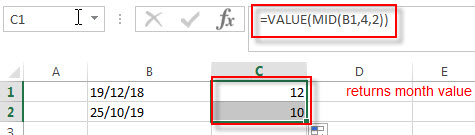The MID function will retrieve a substring from a text string at the position (start_num is 4, and num_chars is 2) in Cell B1, so it will extract two characters at the string position 4. And it will be convert to a numeric value, this value goes into the DATE function as its month argument.

=VALUE(LEFT(B1,2))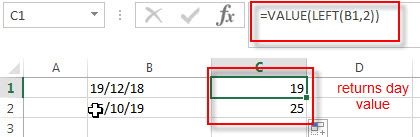The LEFT function will extract the leftmost 2 characters in Cell B1, then convert it as a numeric value by VALUE function. The returned value goes into the DATE function as its day argument.

So far, we got all three arguments (year, month, day) of DATE function from the above formulas, and then we can use the DATE function to convert it as the standard date format. Using the below formula:

=DATE(VALUE(RIGHT(B1,2)+2000), VALUE(MID(B1,4,2)), VALUE(LEFT(B1,2)))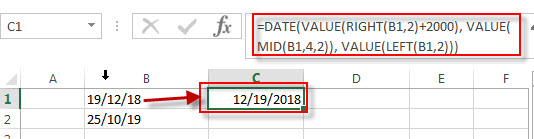You can drag fill handle over other cells which need to use this formula to convert dates in the text format to a date format mm/dd/yyyy.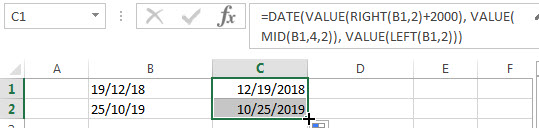Actually, you do not need to use the VALUE function in this formula because excel will convert text to numbers as necessary automatically. So we can write down the below formula:

`=DATE(RIGHT(B1,2)+2000, MID(B1,4,2), LEFT(B1,2))`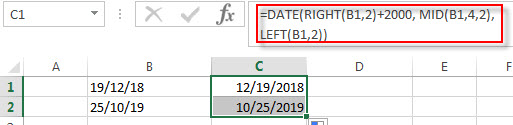### Related Formulas

• Convert date to month name with Text Function
If you want to convert the date to a month name, you can use the TEXT function with a specified format code like “mmm”in excel.  You can try to run the following TEXT formula:=TEXT(B1,”mmm”)
• Convert Date to text with Text Function in Excel
you can use TEXT function in excel to convert dates to text in a specific format code. For example, The TEXT function can use the following patterns, like as: “mm/dd/yyyy”, “yyyy/mm/dd”, etc.
• Convert date to month and year only in excel
If you want to convert the date to month and year only, you can use “yyyymm” format code within the TEXT function in excel, so you can write down the below TEXT formula:=TEXT(date,”yyyymm”)
• Convert date to month and day only in excel
If you want to convert the date (mm/dd/yyyy) to month and day only, you can use “mm dd” format code within the TEXT function in excel, so you can write down the below TEXT formula: =TEXT(date,”mm dd”)

### Related Functions

• Excel DATE function
The Excel DATE function returns the serial number for a date.The syntax of the DATE function is as below:= DATE (year, month, day)…
• Excel MID function
The Excel MID function returns a substring from a text string at the position that you specify.The syntax of the MID function is as below:= MID (text, start_num, num_chars)…
• Excel LEFT function
The Excel LEFT function returns a substring (a specified number of the characters) from a text string, starting from the leftmost character.The LEFT function is a build-in function in Microsoft Excel and it is categorized as a Text Function.The syntax of the LEFT function is as below:= LEFT(text,[num_chars])…
• Excel VALUE function
The Excel VALUE function converts a text value that represents a number to a numeric value.The syntax of the VALUE function is as below:= VALUE  (text)…
• Excel RIGHT function
The Excel RIGHT function returns a substring (a specified number of the characters) from a text string, starting from the rightmost character.The syntax of the RIGHT function is as below:= RIGHT (text,[num_chars])…

## Excel Value Function

This post will guide you how to use Excel VALUE function with syntax and examples in Microsoft excel.

## Description

The Excel VALUE function converts a text string that represents a number to a numeric value.

The VALUE function is a build-in function in Microsoft Excel and it is categorized as a Text Function.

The VALUE function is available in Excel 2016, Excel 2013, Excel 2010, Excel 2007, Excel 2003, Excel XP, Excel 2000, Excel 2011 for Mac.

## Syntax

The syntax of the VALUE function is as below:

`= VALUE (text)`

Where the VALUE function argument is:

• Text-This is a required argument. The text string that you want to convert it to a number.

Note:

• Text can be in any of the constant number, date, or time formats. And if text is not a number, the VALUE function will return #VALUE! Error.
• Normally, you do not need to use the VALUE function in a formula, because Excel converts text string to numeric value as necessary automatically. It is provided for compatibility with other spreadsheet programs.

## Excel VALUE Function Example

The below examples will show you how to use Excel VALUE function to convert string to a number.

#1 To convert a text in B3 cell to a number, just using formula:

`= VALUE(B3)`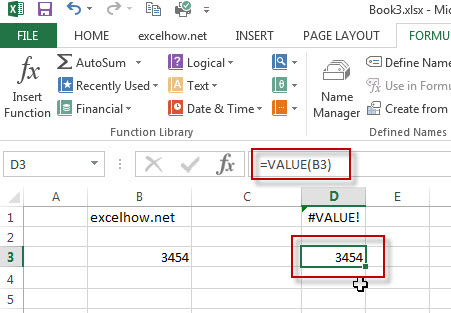#2 to convert a text string that is not a recognized format (number, date, time format) into a numeric value, using the below formula:

`=VALUE(B1)`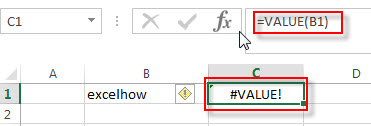You will see that the #VALUE! Error will be returned by the VALUE function. Because the text is not a number.

### More Excel VALUE Formula Examples

• Convet Text Date dd/mm/yy to mm/dd/yyyy as Date Format
If you want to convert dates in the text format to the date format as mm/dd/yyyy, you can use a combination of the DATE function, the VALUE function, the RIGHT function, the MID function, and the LEFT function to create a new excel formula…
• Remove Apostrophe or Text Indicator
If you want to remove the text indicator (‘) or the leading Apostrophe from a text string in one cell, you can create a formula based on the VALUE function….